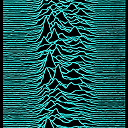Score:2Crypto

# Linear Complexity of two dimensional finite patterns such as QR codesTwo dimensional patters are omnipresent in information transactions. QR codes, images are most common. I want to know if there is a concept analogous to the well known concept of Linear Complexity of periodic sequences, for two dimensional patterns?

Score:3CryptoTwo dimensional linear recurrences are a bit more complex algebraically.

The concept corresponding to the linear complexity would in some sense be the degree $$(n,m)$$ of the minimal degree generating polynomial of the form $$x^{n}y^m- \sum_{0\leq i\leq n-1} \sum_{0\leq j\leq m-1} a_{i,j} x^i y^j$$ which would correspond to the 2D linear recurrence $$s_{t+n,t'+m}=\sum_{0\leq i\leq n-1} \sum_{0\leq j\leq m-1} a_{i,j} s_{t+i,t'+j}$$ where $$t,t'$$ are the indices in the two dimensions.

Sakata has generalised the Berlekamp Massey algorithm (BMA) to 2, and then to N dimensions; See here (seems to be publicly available). From the abstract:

We present an algorithm for finding a minimal set of two-dimensional linear recurring relations capable of generating a prescribed finite two-dimensional array. This is a two-dimensional extension of the Berlekamp-Massey algorithm for synthesizing a shortest linear feedback shift-register capable of generating a given finite sequence. The complexity of computation for an array of size $$n$$ is $$O(n^2)$$ under some reasonable assumptions. Furthermore, we make clear some relationship between our algorithm and Gröbner bases of bivariate polynomial ideals, where polynomials correspond one-to-one to linear recurring relations.

The reason they are complex is that (up to some handwaving) for a finite field $$\mathbb{F}$$ the one variable polynomial ring $$\mathbb{F}[x]$$ all ideals are principal (have a single polynomial generator). So for the BMA to work it is sufficient to find one generator of minimum degree. In multiple variables, not all ideals of $$\mathbb{F}[x_1,\ldots,x_n]$$ are principal.

The definition of two variable degree and the ordering of degrees of terms is another issue, but that can be done via lexicographic ordering for example.Thank you very much Kodlu. This has given me a good start on my problem.